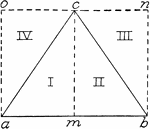### Area of Triangle

"The triangle ABC is divided into 2 right triangles I and II. ABC is seen to be equal to 1/2 of the…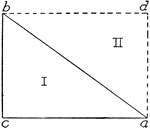### Area of Triangle

"The child sees triangle ACB = triangle ADB, and that I + II = CA - DB; and so he sees that the area…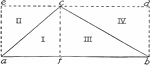### Area of Triangle

"Fig. 3 shows triangle I = triangle II, III = IV, and so triangle ABC = 1/2 of rectangle ABDE. The fact…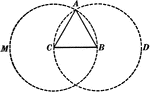### Construction Of Equilateral Triangle

Illustration used to show how to construct an equilateral triangle, with a given line as a side.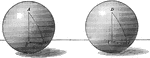### Equal Spheres With Triangles

Illustration of equal spheres with equal triangles.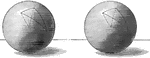### Equal Spheres With Triangles

Illustration of equal spheres with equilateral and equiangular triangles.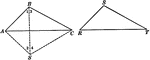### Equal Triangles

Illustration used to show that two triangles are equal if the three sides of one are equal respectively…